>

## Chapter 7 Statistics R.D. Sharma Solutions for Class 10th Math Exercise 7.4

1. Following are the lives in hours of 15 pieces of the components of aircraft engine. Find the median:
715, 724, 725, 710, 729, 745, 694, 699, 696, 712, 734, 728, 716, 705, 719.

Solution2. The following is the distribution of height of students of a certain class in a certain city:
Height (in cm): 160-162 163-165 166-168 169-171 172-174
No. of students:  15  118 142 127 18
Find the median height.

Solution3. Following is the distribution of I.Q. of loo students. Find the median I.Q.
I.Q.:   55-64  65-74  75-84  85-94  95-104  105-114  115-124  125-134  135-144
No of Students: 1  2  9  22  33  22  8  2  1

Solution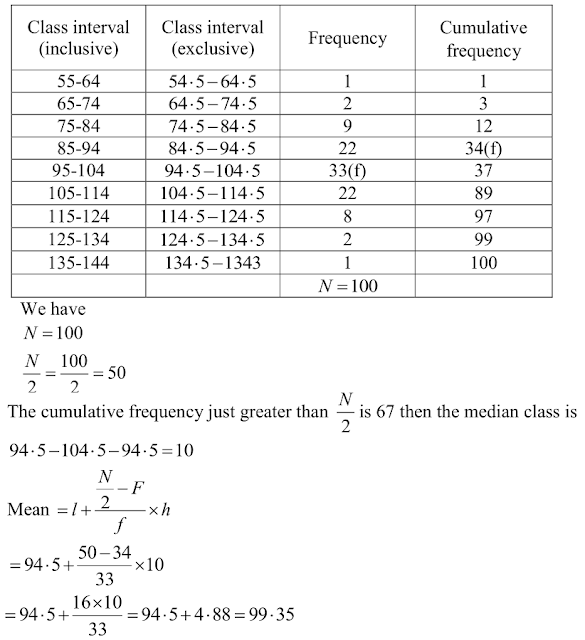4. Calculate the median from the following data:
Rent (in Rs.): 15-25 25-35 35-45 45-55 55-65 65-75 75-85 85-95
No. of Houses: 8  10  15  25  40  20  15  7

Solution5. Calculate the median from the following data:
Marks below: 10 20 30 40 50  60   70 80
No. of students: 15 35 60 84 96 127 198 250

Solution6. Calculate the missing frequency from the following distribution, it being given that the median of the distribution is 24.
Age in years:  0-10 10-20 20-30 30-40 40-50
No. of persons: 5  25  ?  18  7

Solution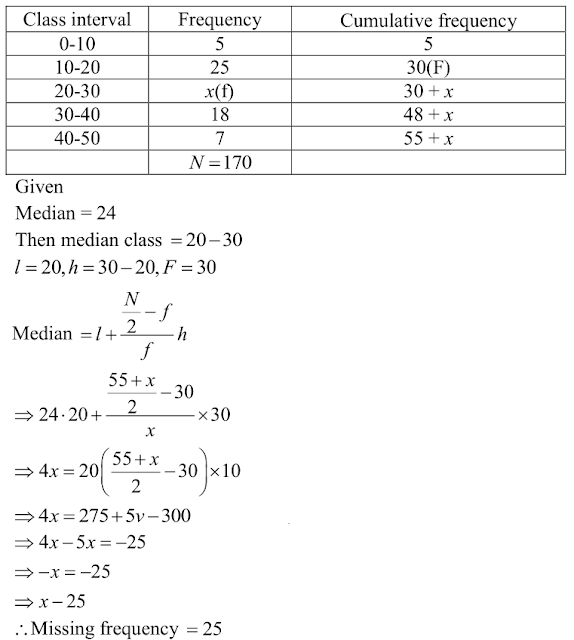7. The following table gives the frequency distribution of married women by age at marriage:Calculate the median and interpret the results

Solution8. The following table gives the distribution of the life time of 400 neon lamps: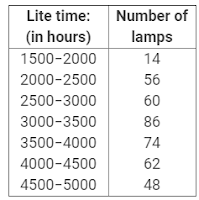Find the median life .

Solution9. The distribution below gives the weight of 30 students in a class. Find the median weight of students:
Weight (in kg): 40-45 45-50 50-55 55-60 60-65 65-70 70-75
No. of students:  2  3  8  6  6  3  2

Solution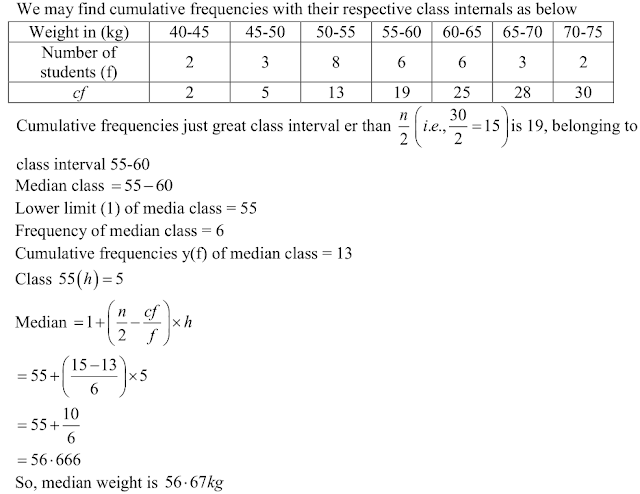10. Find the missing frequencies and the median for the following distribution if the mean is
1.46.
No. of accidents:  0  1  2  3   4  5  Total
Frequency (No. of days): 46  ?  ?  25  10  5  200

Solution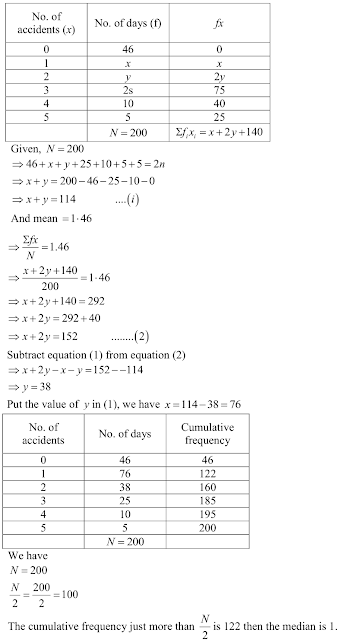11. An incomplete distribution is given below:
Variable: 10-20  20-30  30-40  40-50  50-60  60-70  70-80
Frequency: 12  30  -  6  5 -  25   18
You are given that the median value is 46 and the total number of items is 230.
(i) Using the median formula fill up missing frequencies.
(ii) Calculate the AM of the completed distribution.

Solution

(i)(ii)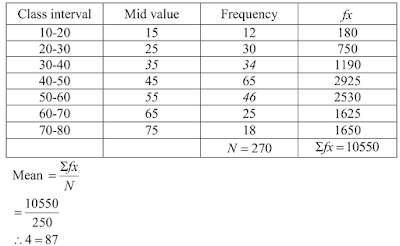12. If the median of the following frequency distribution is 28.5 find the missing frequencies:
Class interval: 0-10 10-20 20-30 30-40 40-50 50-60 Total
Frequency:         5        f 1     20       15     f 2      5      60

Sol.13. The median of the following data is 525. Find the missing frequency, if it is given that there are 100 observations in the data: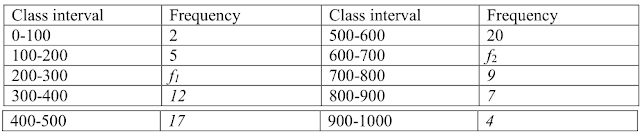Solution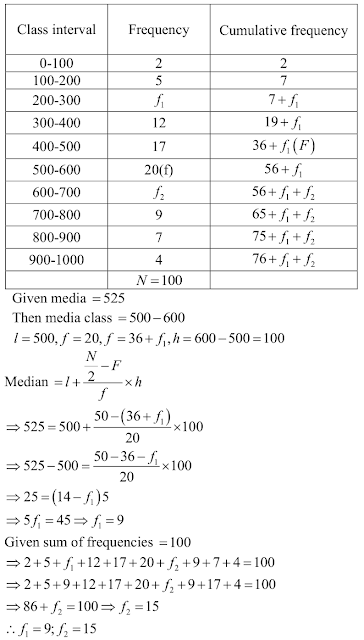14. If the median of the following data is 32.5, find the missing frequencies.
Class interval: 0- 10  10-20  20-30  30-40  40-50  50-60  60-70  Total
Frequency: f1  5  9  12  f2  3  2  40

Solution15. Compute the median for each of the following data:
(i)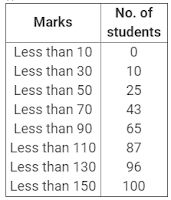(ii)Solution

(i)(ii)16. A survey regarding the height (in cm) of 51 girls of class X of a school was conducted and
the following data was obtained:
Height in cm  Number of Girls
Less than 140   4
Less than 145  11
Less than 150  29
Less than 155  40
Less than 160  46
Less than l65   51

Find the median height.

Solution

To calculate the median height, we need to find the class intervals and their corresponding frequencies .
The given distribution being of thee less than type 140, 145, 150,…..,165 give the upper limits of the corresponding class intervals. So, the classes should be below 140, 145, 150,….., 160, 165 observe that from the given distribution, we find that there are 4-girls with height less than 140 is 4. Now there are 4 girls with heights less than 140. Therefore, the number of girls with height in the interval 140, 145 is 11- 4=7, similarly. The frequencies of 145 150 is 29-11=18, for 150-155 it is 40-29=11, and so on so our frequencies distribution becomes.17. A life insurance agent found the following data for distribution of ages of 100 policy holders. Calculate the median age, if policies are only given to persons having age 18 years onwards but less than 60 years.
Age in years  Number of policy holders
Below 20   2
Below 25   6
Below 30   24
Below 35   45
Below 40   78
Below 45   89
Below 50   92
Below 55   98
Below 60   100

Solution18. The lengths of 40 leaves of a plant are measured correct to the nearest millimeter, and the data obtained is represented in the following table:
Length (in mm): 118-126  127-135  136-144  145-153  154-162  163-171  172-180
No. of leaves:  3   5   9  12   5  4  2
Find the mean length of life.

Solution19. An incomplete distribution is given as follows:
Variable: 0-10 10-20  20-30  30-40  40–50  50-60  60-70
Frequency: 10  20  ?  40  ?  25 15
You are given that the median value is 35 and the sum of all the frequencies is 170. Using
the median formula, fill up the missing frequencies.

Solution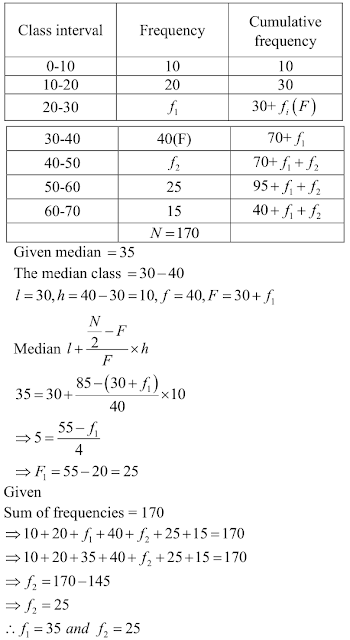20. The median of the distribution given below is 14.4 . Find the values of x and y , if the total frequency is 20.
Class interval : 0-6  6-12 12-18 18-24 24-30
Frequency :        4       x       5        y       1

Solution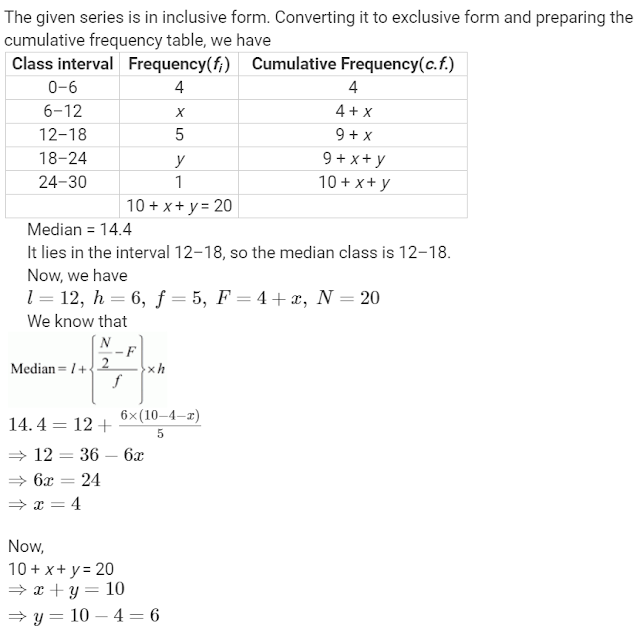21.  The median of the following data is 50. Find the value of p and q , if the sum of all the frequencies is 90.

Solution